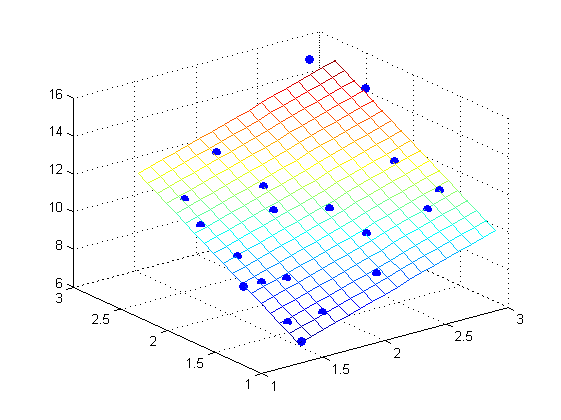# 2.代码设计

% 随机生成一组（x,y,z),这些点的坐标离一个空间平面比较近
x0=1,L1=2;
y0=1,L2=2;
x=x0+rand(20,1)*L1;
y=y0+rand(20,1)*L2;
z=1+2*x+3*y;
scatter3(x,y,z,'filled')
hold on;

planeData=[x,y,z];

% 协方差矩阵的SVD变换中，最小奇异值对应的奇异向量就是平面的方向
xyz0=mean(planeData,1);
centeredPlane=bsxfun(@minus,planeData,xyz0);
[U,S,V]=svd(centeredPlane);

a=V(1,3);
b=V(2,3);
c=V(3,3);
d=-dot([a b c],xyz0);

% 图形绘制
xfit = min(x):0.1:max(x);
yfit = min(y):0.1:max(y);
[XFIT,YFIT]= meshgrid (xfit,yfit);
ZFIT = -(d + a * XFIT + b * YFIT)/c;
mesh(XFIT,YFIT,ZFIT);

# 3.实验结果09-1904-02
05-29
09-101万+
11-101万+
05-137671
12-232万+
05-063630
10-15
08-204603
12-21
07-093593
01-051万+
04-062872
03-124755
11-236092
12-01450
03-221万+